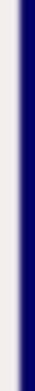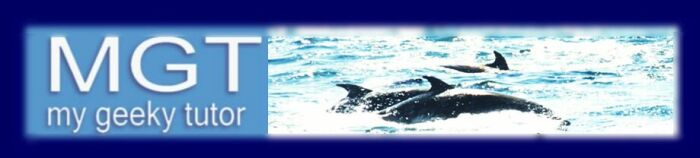homework help forum today's math problem links utilities previous math problem feedback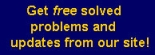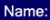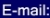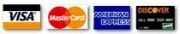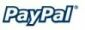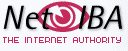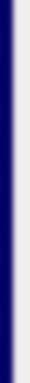# MATH HOMEWORK HELP

10. A firm’s total cost of producing q units is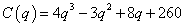.

The current level of production is four units. Using incremental change (also called the differential), estimate the amount by which the total cost will change as a result of increasing production by 1/8 unit.

Solution: The differential is defined as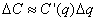Notice that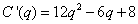Therefore, the estimated amount by which the total cost will change is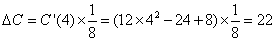MGT - Your source of Math and Statistics Homework Help